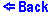Kirchhoff's Rules (Circuit 5)

Increase/decrease the magnitude of the EMFs and the resistors by clicking on the upper/lower part of the object. The values of the resistors range from 1 to 10 ohms. And the values of the EMFs range from -10 to +10 Volts. The ammeters show the currents in the wires, the voltmeters show the voltages across the resistors.
This is the most compicated of all cases covered. The circuit consists of a loop ABCDEF and the following components attached to each of it's three branches: an EMF, an Ammeter which measures the current through that branch, a resistor, and a Voltmeter which measures the potential rise/drop on the resistor. The values for each of the components can be seen either next to or written on the components themselves. It should be made clear that the Ammeters and Voltmeters are positioned in such a way that they will show a positive reading when the current is flowing from top to bottom, although such a selection is made in this circuit it would have been just as correct to pick it's opposite.

These three basic rules/formulas are needed to solve the system of equations envolved:

• 1. Kirchhoff's Junction Rule,
• 2. Kirchhoff's Loop Rule and
• 3. Ohm's Law (i.e. V/I = R,
• where I is the current, V is the voltage, and R is the resistance).

To solve the equations we will need to name our components, we have chosen a system in which each component is named by a letter indicating it's type (I for current, E for the EMF, and R for resistance) and a subletter indicating it's branch (A, B, or C). Thus the current in the leftmost branch would be (by Ohm's Law): IA = VA/RA. Now we are ready to formulate the system.

First, we take the outer most loop ACDF and use the Junction Rule. Let's take junction B, since we assume that all of the currents flow from top to bottom, the sum of the currents heading out of the loop is equal to zero (since no current is heading in) i.e.,
IA + IB + IC = 0.

Next we take loop ABEF and apply the Loop Rule, which says that the sum of the potential rises must equal to the sum of the potential drops i.e.,
EA + IA*RA = EB + IB*RB;

we also apply this rule to the outer loop, ACDF, getting:
EA + IA*RA = EC + IC*RC.

Let us summarize our system of three equations:
IA + IB + IC = 0;
EA + IA*RA = EC + IC*RC;
EA +IA*RA = EB + IB*RB;

Solving for the currents we get:
IB = (EA - EB )/RB + IA*(RA/RB);
IC = (EA - EC)/RC + IA*(RA/RC);
IA = ((EB - EA)/RB + (EC - EA)/RC) / (1 + (RA/RB ) + (RA/RC));

Therefore, since the resistance, R, and the EMF, E, are known for each of the branches, the currents, I, are calculated by the above method.

The voltage across the resistors is arrived at as follows: (let V be the voltage across the resistor, by Ohm's Law)
V = IR;

Then we substitute any of the above values for I.

Example, VB = IB*RB = RB*((EA - EB)/RB + IA*(RA/RB)).